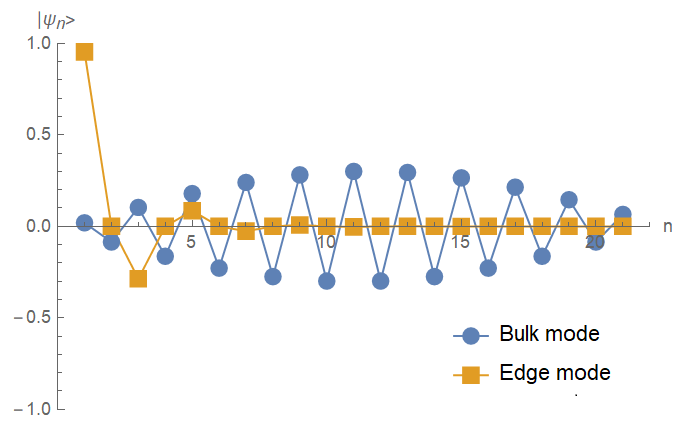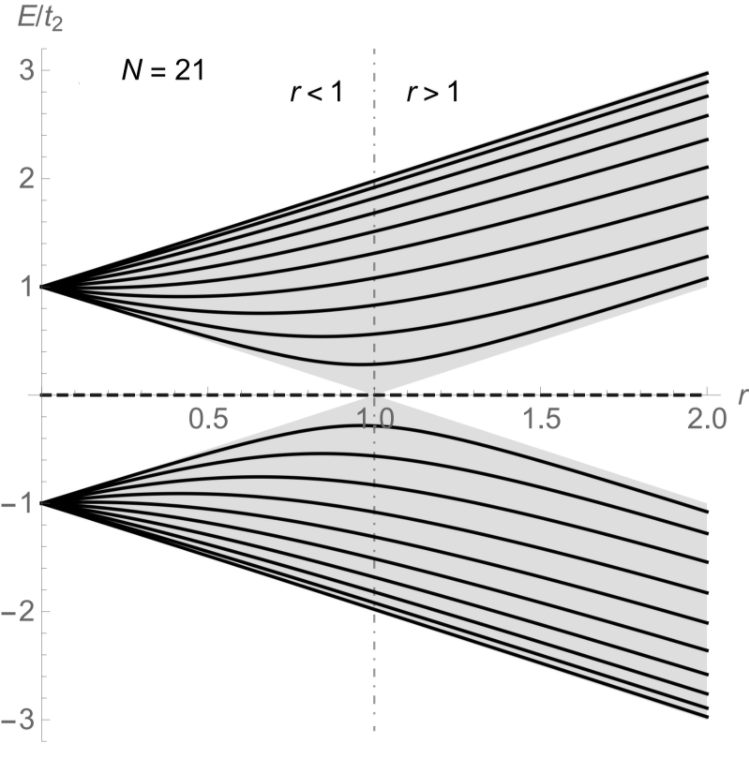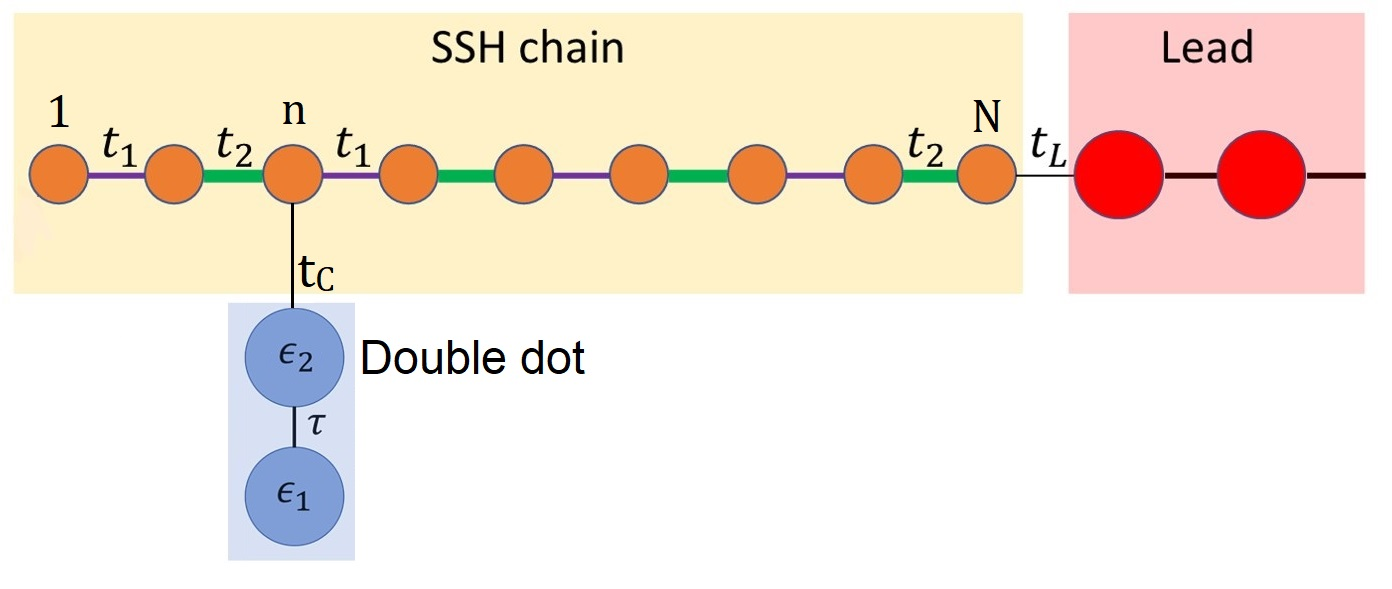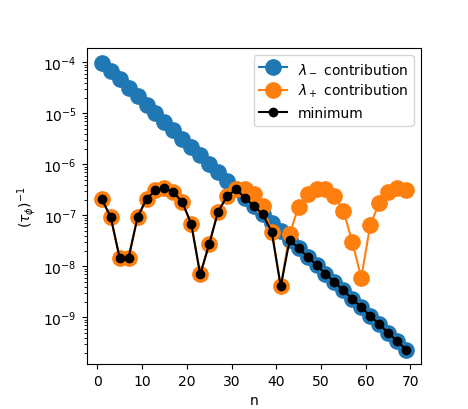# Qubits as Probes for Topological Materials

## A study of decoherence

Nicolas Delnour1, Richard MacKenzie1, Michael Hilke2, Hichem Eleuch3
Université de Montréal1, McGill University2, University of Sharjah3

### Introducing Concepts

Topological insulator (TI) TIs can be defined as materials acting as insulators in the bulk while hosting symmetry protected topological (SPT) states at their boundaries.
• A simple TI model: The Su-Schrieffer-Heeger (SSH) model describes the transport of a fermion along a dimerized chain .
• The SSH model can host 0, 1, or 2 edge (SPT) states based on its topological phase and parity.Fig. 2 - Comparison of a bulk vs edge solutions to the Schrödinger equation for an SSH chain of length N=21.
• The edge states are of zero or near-zero energy, as can be seen with the spectrum below.Fig. 3 - Energy spectrum as a function of hopping parameter ratio r for N=21. Solid (dashed) lines are the bulk (edge) energies. The shaded grey region shows energy bands in the thermodynamic limit . Qubit

A qubit or double dot (DD) is a two-level system (TLS) in a coherent superposition of two states. The Hamiltonian for a qubit is expressed simply as $H^{D D}=\left(\begin{array}{cc} \epsilon_{1} & \tau \\ \tau & \epsilon_{2}\end{array}\right)$ with eigenenergies λ ±. The transition probability between both levels can be found from the off-diagonal element of the Green's function G12 with respect to time: $G^{\mathrm{DD}}(E)=(E-H^{\mathrm{DD}})^{-1}$ $G_{12}^{\mathrm{DD}}(t)=\int_{-\infty}^{\infty} d E e^{-i E t} G_{12}^{\mathrm{DD}}(E)$

• Isolated qubit: Solutions to G12(t) are simple periodic oscillations.
• Qubit in open system: Solutions to G12(t) are decaying oscillations. Decaying solutions imply that the 1 ⇄ 2 transition probability goes to zero as the qubit interacts with its environment; decoherence in an open system can therefore be evaluated by finding G12(t).

### Tripartite System

We consider an open system shown in Figure 4 consisting of a qubit coupled (coupling strength tc) to an SSH chain to which is, in turn, coupled a semi-infinite lead (coupling strength tL).Fig. 4 - Tripartite system including a qubit, SSH chain, and semi-infinite conducting lead. The qubit is coupled at an arbitrary nth site.

The Hamiltonian for such a system has the form: $H=\left(\begin{array}{c|c|c} H^{DD} & V_N & 0 \\ \hline V_N^{\dagger} & H_{\mathrm{SSH}} & W \\ \hline 0 & W^{\dagger} & H_{\infty} \end{array}\right)$

As we are interested in the behaviour of the qubit, we can incorporate the impact of the environment into a self-energy term  such that: $H^{D D \prime}=\left(\begin{array}{cc} \epsilon_{1} & \tau \\ \tau & \epsilon_{2}+\Sigma_{\mathrm{SSH},\infty}^{nn}(E) \end{array}\right)$ where $\Sigma_{\mathrm{SSH},\infty}^{nn}(E) = t_{c}^{2} G_{\mathrm{SSH},\infty}^{nn}(E)$

• The self-energy's non-trivial dependence on the energy renders the Fourier transform for the time-dependent Green's function impossible.
• Analytical approximation: for small couplings between the qubit and the system, it is valid to evaluate the qubit frequencies λ' ±(E) at the poles of the isolated qubit λ ± . This effectively removes the problem of the self-energy in the Fourier transform.
• A decay rate can now be evaluated according to the analytical approximation above. One finds:

$\left(\tau_{\phi}\right)^{-1} \approx \min \left(-\frac{1}{2} \Im\left\{\Sigma^{nn}_{\mathrm{SSH}, \infty}\left(\lambda_{\pm}\right) \pm \delta^{\prime}\left(\lambda_{\pm}\right)\right\}\right)$

• Above, min stands for minimum and selects the rate dominating at long times; the slowest decay is representative of the decoherence of the qubit .

### Proposed Measurement

• Initialise qubit such that λ- resides at the midgap of the SSH bands (i.e. tune qubit energies to energy of the edge state).
• Couple to site n to the SSH chain and measure decoherence.
• Translate to site n+1 and repeat above steps.

This sweep through the SSH chain allows us to characterise the decoherence as a function of site number for a given qubit tuning.Fig. 5 - Decoherence rate of a qubit coupled to the SSH chain (length N = 69 with single left edge state) and lead system. Note two different regimes: 1) oscillatory behaviour associated to coupling with bulk states from λ+= 0.4044. 2) exponential decay from coupling of edge state with λ-= 0. Information gained
• The qubit couples strongly with states in the SSH chain with energies close to λ ±. Tuning a qubit to have λ- = 0 therefore couples one of the frequencies strongly with the edge state.
• Stronger coupling to the environment implies quick decoherence for the qubit and allows for the identification of the presence/location of gapped SPT states.
• Additionally, the slope of the λ-= 0 contribution to the decoherence (ɑdecay) is directly linked to the physical amplitude of the edge state:

$e^{\alpha_{decay} n} \sim |\psi_{edge} |^2$

### Discussion and future avenues for research

• Qubits can detect topological edge states and infer which boundary the states lie on via a study of decoherence dynamics.
• The decoherence dynamics additionally provide a direct tool for observing the squared magnitude of the topological modes thus allowing for the observation of a very fundamental quantity.
Where to look from here?
• A better understanding of the qubit's bulk coupling oscillations may lead to rich physical behaviours.
• Domain walls / solitons / defects between topological phases also host midgap states which are expected to behave similarly to edge states when probed by a qubit.
Why care? This analysis considers a "toy-system" in order to evaluate decoherence of an open system within reasonable analytical approximations, but qubit behaviours should extend to more complex systems. Qubits could therefore be useful for:
• Characterisation of topological excitations in more complex topological insulators, but also in other materials such as superconductors.
• Characterisation of domain walls and defect modes in solids.
• Certain materials exhibit interesting topological modes that are Majorana fermions. These have been proposed to have important applications in quantum computing and this probing method could be used to deepen our understanding of these exotic excitations.
• References
 A.J. Heeger, S. Kivelson, J.R. Schrieffer, and W.-P. Su, Rev. Modern Phys.60, 781 (1988)
 J.K. Asboth, L. Oroszlany, A. Palyi, A short course on topological insulators, Lecture Notes in Physics 919 (Springer Int. Pub., 2016)
 Zaimi, M., Boudreault, C., Baspin, N., Delnour, N., Eleuch, H., MacKenzie, R., Hilke, M. (2021) Phys. Lett. A. 388 : 127035
 S. Datta,Quantum transport: Atom to Transistor, (Cambridge University Press, 2005)
 H. Eleuch, M. Hilke, R. MacKenzie, Physical Rev. A95, 062114 (2017)